# Essentials Of Cost Accounting

## Quiz 6 : Cost-Volume-Proﬁt AnalysisStudy FlashcardsLooking for Accounting Homework Help?

## Quiz 6 :Cost-Volume-Proﬁt Analysis

Question TypeWhat are some assumptions underlying breakeven analysis that require consideration by a manager employing the technique?
Free
Essay

Breakeven analysis:
It is a managerial tool that is based on the cost, volume and profit. It determines required sales volume and dollar sales to compensate for the given cost structure or price level. At break-even point, total revenue is equal to the total cost. Hence, this is the situation of no profit, no loss. Above break-even point, company starts making profit. In other words, break-even point determines minimum revenue to be generated to recover the cost of production.
Some of the assumptions with respect to breakeven analysis that the manager should consider are listed below:
1. Before starting breakeven analysis all costs should be separated into fixed and variable components.
2. At all volumes, fixed costs should remain constant.
3. Before starting breakeven analysis, it should be assumed that price for different types of patients and for different payers will be constant as volume changes.
4. In order to calculate breakeven, manager needs to estimate the average amount per patient which would be collected from the patients.
5. It is assumed that variable costs will remain same as they are.

Tags
Choose question tag(Note: This problem has been adapted from an earlier problem presented by Horngren,* which came originally from a CPA exam.) Aspen Hospital allocates overhead costs to departments such as pediatrics, maternity, psychiatric, and so forth. Aspen charges each separate department for common services such as meals and laundry and for administrative services such as billings and collections. For the year ended June 30, 2007, the operating room department at Aspen Hospital charged each patient an average of $1,650 per operation and had revenue of$2,656,500. Expenses charged by the hospital to the operating room department for the year ended June 30,2007, were as follows: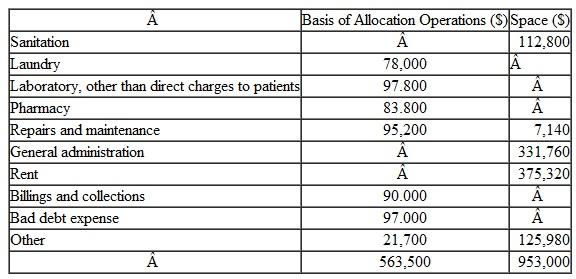The only personnel directly employed by the operating room are supervising nurses, scrub nurses, and circulating nurses. The hospital has minimum personnel requirements based on total operations. Hospital requirements beginning at the minimum expected level of operations follow: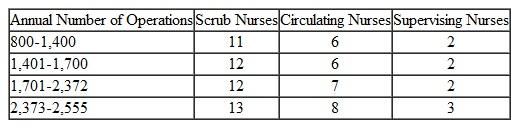The staffing levels above represent fulltime equivalents, and it should be assumed that the operating room always employs only the minimum number of required fulltime equivalent personnel. Annual salaries for each class of employee follow: supervising nurses, $68,500; scrub nurses,$59,000; and circulating nurses, $54,000. The operating room has capacity for only 2,000 operations per year at the current time. Calculate the minimum number of operations required for the operating room to break even for the year ending June 30, 2008. Patient demand is unknown, but assume that revenue per operation and costs remain at the same rates as last year. Free Essay Answer: Answer: Break-even analysis It determines the quantity at which the revenue will be equal to the cost incurred. In other words, it determines the quantity at which there is no profit and no loss. It can be understood as the level of sales at which company is able to recover all the expenses and start earning the profit. It can be calculated by given formula: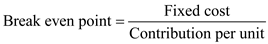In the given problem, the overhead details of operating room department of A are given for the year 2007. Its total revenue is$2,656,500. It charges each patient $1,650 per operation. The hospital has also charged some overheads to operating room department which amounts to total of$1,516,500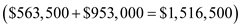. This is not related to number of operations performed by the department in any way. Therefore, it can be considered as fixed cost to the department.
The department has 3 types of employees depending on the number of operations to be performed annually. This is the variable cost to the department.
To calculate the break-even point it is required to consider every range of operations separately.
For 800 to 1,400 operations
The given table shows information's about above range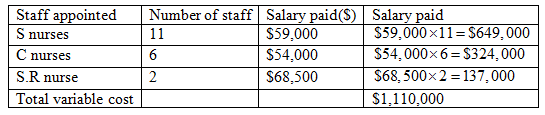Variable cost is $1,110,000 Fixed cost is$1,516,500
Therefore,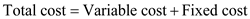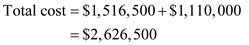At break-even point,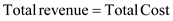Where,
Q is the number of patients required to break even
And cost charged to each patient is revenue for hospital.(Given in question)
Breakeven point is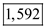This means that to achieve break-even point, the department has to perform 1,592 operations. But this number is out of the given range.
Therefore, it can be concluded that 1,592 is not the feasible break-even point.
1,401 to 1,700 operations
The given table shows information's about above range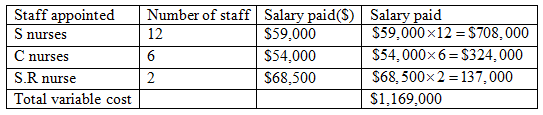Therefore, variable cost = $1,169,000 As calculated above, fixed cost is$1,516,500
Therefore,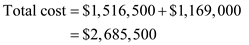At break-even point,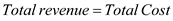Breakeven point is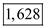This means that to achieve break-even point, the department has to perform 1,628 operations. This number is within the given range.
Therefore, it can be concluded that this is can be the feasible break-even point.
1,701 to 2,372 operations
The given table shows information's about above range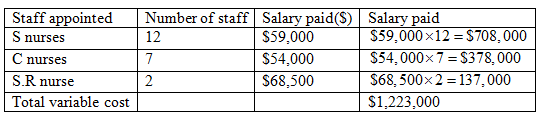Therefore, variable cost is $1,223,000 As calculated above, fixed cost is$1,516,500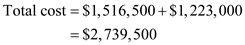At break-even point,Breakeven point is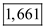This means that to achieve break-even point, the department has to perform 1,661 operations. But this number is out of the given range.
Therefore, it can be concluded that it is not the feasible break-even point.
2,373 to 2,555 operations
The given table shows information's about above range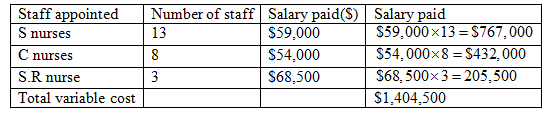Therefore, variable cost is $1,404,500 As calculated above, fixed cost is$1,516,500
Therefore,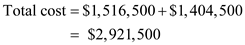At break-even point,Breakeven point is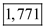This means that to achieve break-even point, the department has to perform 1,771 operations. But this number is out of the given range.
Therefore, it can be concluded that it is not the feasible break-even point.
Thus, from above calculations it can be concluded that that the department should perform minimum 1,628 operations to break even in 2008.

Tags
Choose question tagStellar Outpatient Clinic has the following patient mix: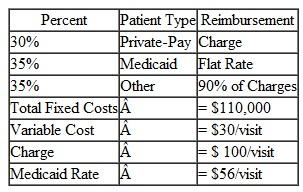You want the clinic to earn a $100,000 profit this year. What breakeven volume is necessary for this to occur? Free Essay Answer: Answer: Breakeven point (BEP) is the level where the revenue earned equals the cost of earning such revenue. Thus, it is situation where the firm is earning no profit as well as incurring no losses. Thus, BEP is that point where the income equals the invested cost. The formula to calculate BEP is: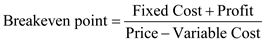There table presents the facts of the query. There are three types of visitors that visit in the clinic each has different rate. The Private type patient are charged$100 per visit, Medicaid are charged $56 per visit, others are charged 90% of private type patient that is 90% of$100 which amounts to $90.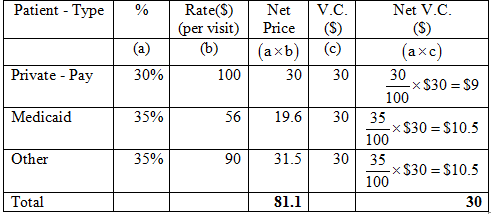Here, per visit rate (column b) is multiplied with the percent of patients (column a) to determine the weighted price. Similarly the variable cost (column c) is multiplied to calculate the weighted variable cost per unit. This assists in ascertaining the value of BEP admissions that will incur$100,000 profit for the clinic.
Calculate BEP when the total fixed cost is $110,000, profit desired is$100,000 andThus,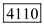patients should be admitted to have a profit of $100,000. Tags Choose question tagElite Hospital has$400,000 of fixed overhead costs. It has the following three types of patients: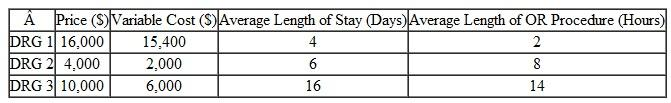When Elite Hospital plans its new marketing campaign, what type of patient should they try to attract, assuming: a) They are not near capacity in any area. b) They are nearly full and can take a limited number of additional patient-days. c) They have plenty of beds but are running out of operating room time.
Essay
Tags
Choose question tagTrue or false: As long as the revenue per patient is at least equal to the variable cost per patient, the service or program will break even.
True False
Tags
Choose question tagTrue or false: Average costs fall as volume rises, as a result of fixed costs.
True False
Tags
Choose question tagTrue or false: It is essential that breakeven analysis always consider all costs related to a program or service, including the joint costs.
True False
Tags
Choose question tagThe Heal Up Clinic provides annual checkups for a charge of $200, sick visits for$100, and flu shots for $40. They expect to have 500 checkups, 1,000 sick visits, and 400 flu shots each month. The variable costs re lated to annual checkups are$10, related to sick visits are $5, and related to flu shots are$20. Rent on their medical facilities is $120,000 per year. Other fixed costs for utilities are$5,000 per month. If the owners of the clinic want to at least break even, what is the most they can pay their employees each month?
Essay
Tags
Choose question tagYour Eastlawn Surgery Center is considering adding a new service, which encompasses several types of patients. If you add the service, you will get patients in each of several types for which you do not currently have any admissions. You are given the following data: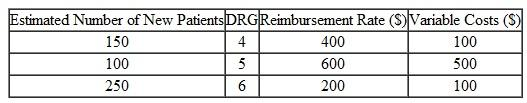There will be no fixed cost specifically associated with any one of the three new types of patients. The fixed costs for the new service that makes up these three types will be $25,000. The joint overhead costs of the surgery center are$500,000, currently being allocated to the surgery c en te r's three existing services. How many admissions in each of the new types of patients will be needed to break even on the new service?
Essay
Tags
Choose question tagWhat is the essence of using breakeven analysis for multiple services and/or multiple prices?
Essay
Tags
Choose question tagWhy is volume considered to be critical for any new venture?
Essay